The PANEL Procedure

Panel Data Poolability Test

Subsections:

The null hypothesis of poolability assumes homogeneous slope coefficients. An F test can be applied to test for the poolability across cross sections in panel data models.

F Test

For the unrestricted model, run a regression for each cross section and save the sum of squared residuals as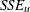. For the restricted model, save the sum of squared residuals as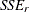. If the test applies to all coefficients (including the constant), then the restricted model is the pooled model (OLS); if the test applies to coefficients other than the constant, then the restricted model is the fixed one-way model with cross-sectional fixed effects. If N and T denote the number of cross sections and time periods, then the number of observations is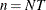. Let k be the number of regressors except the constant. The degree of freedom for the unrestricted model is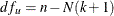. If the constant is restricted to be the same, the degree of freedom for the restricted model is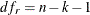and the number of restrictions is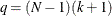. If the restricted model is the fixed one-way model, the degree of freedom is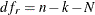and the number of restrictions is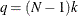. So the F test is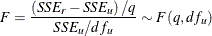For large N and T, you can use a chi-square distribution to approximate the limiting distribution, namely,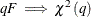. The error term is assumed to be homogeneous; therefore,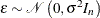, and an OLS regression is sufficient. The test is the same as the Chow test (Chow, 1960) extended to N linear regressions.

LR Test

Zellner (1962) also proved that the likelihood ratio test for null hypothesis of poolability can be based on the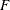statistic. The likelihood ratio can be expressed as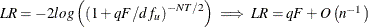. Under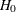,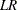is asymptotically distributed as a chi-square with q degrees of freedom.

 For the unbalanced panel, the number of time series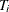might be different. The number of observations needs to be redefined accordingly.